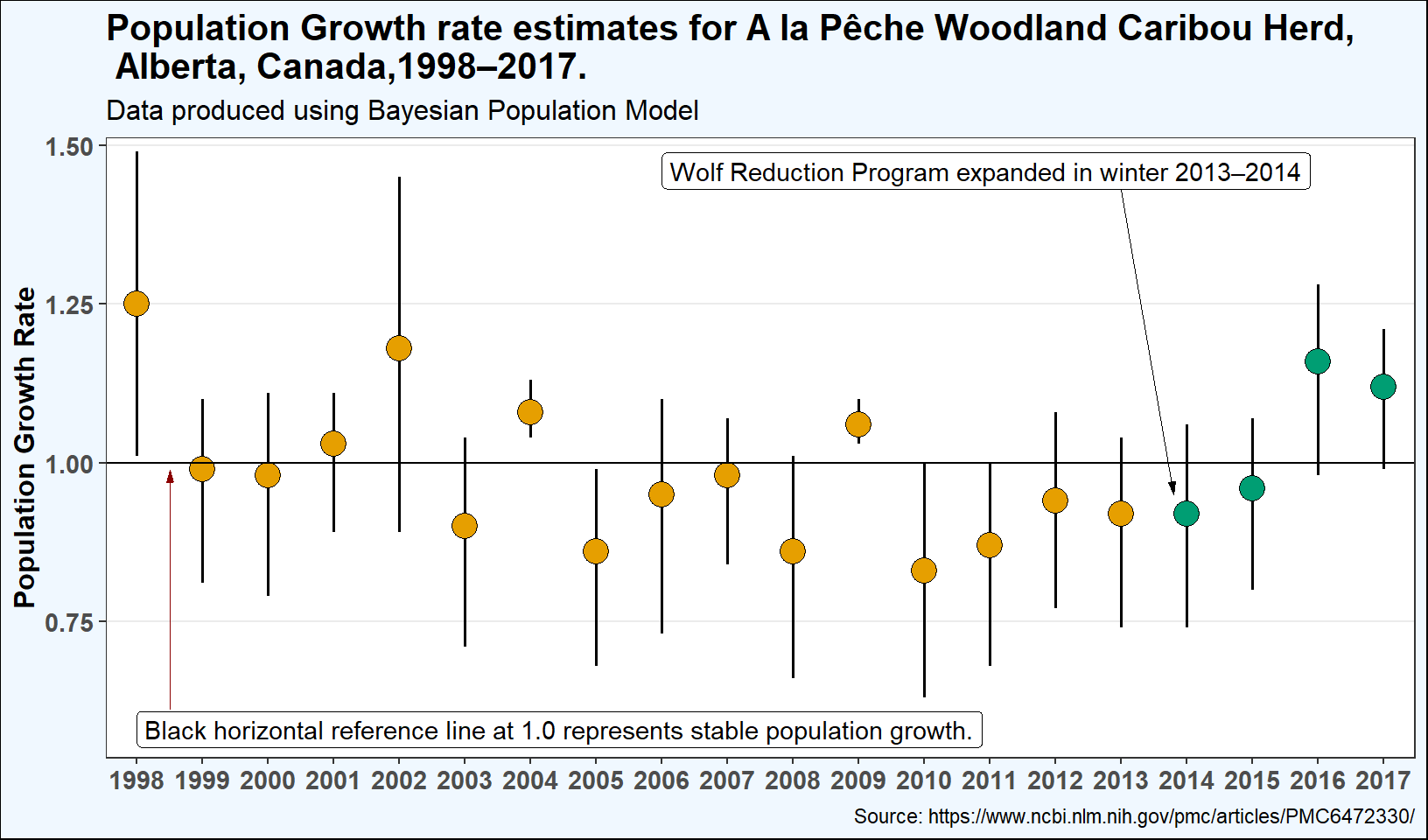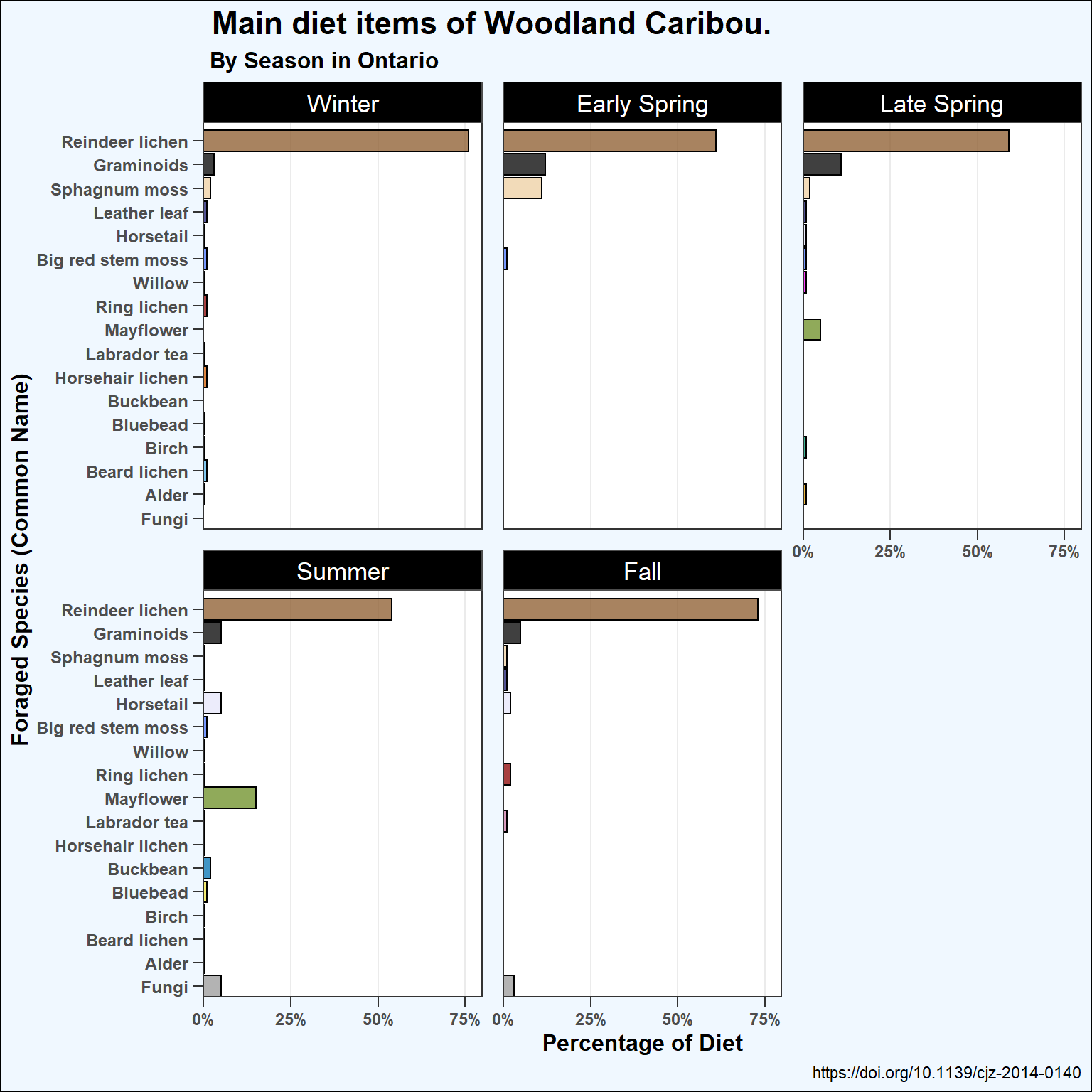# Packages and Data

setwd("~/MTH 250")
library(tidyverse)

# Background

str(ff)

lvls_season <- c("Winter","Early.Spring","Late.Spring","Summer","Fall")

diet <- read.csv("WoodlandCaribouDiet.csv",stringsAsFactors=FALSE) %>%
pivot_longer(cols=-1,names_to="Season",values_to="Proportion") %>%
mutate(CN=trimws(CN,which="right"),
CN=factor(CN),
CN=forcats::fct_reorder(CN,Proportion),
Season=factor(Season,levels=lvls_season))

str(diet)
cbPalette <- c("#999999","#E69F00","#56B4E9","#009E73",
"#F0E442","#0072B2","#D55E00","#CC79A7","olivedrab","darkred",
"magenta","royalblue1","lavender","midnightblue",
"navajowhite2","gray0","tan4")

# Population Growth Rate

The following plot shows the population growth rate estimates for A la Peche Woodland Caribou Population in AB, Canada from 1998-2017. The researchers in this study wanted to test a new Web based application using a Bayesian estimation approach, since Boreal Woodland Caribou have a very low detection rate and are listed as threatened or endangered across Canada. The plot below shows the population staying right above or below the stable population growth line during the first few years, then consistently staying below the stable population growth line except for 2004 and 2009, with most recently rising above the stable population line after expanding the wolf cull program to take place in both the summer and winter ranges of the Caribou Population. The points on the graph represent the mean estimate for the population growth, with the black error bars being the Confidence Intervals. The CI varies due to the fact that this population, along with most Woodland caribou populations, live in remote, boreal ecosystems where the traditional survey technique of fly over estimates is not practical.

I decided to use points and error-bars to represent the data here because you can get a better and more detailed look at how the population growth rate was each individual year, with the colors representing if there was a complete wolf cull on just the winter range or both summer and winter ranges.

C <- ggplot(data=ff,mapping=aes(x=Year,y=X.)) +
geom_errorbar(aes(ymin=Low.P.BCI,max=Up.P.BCI,width=.15),size=.7,width=.001) +
geom_point(mapping=aes(fill=EX.wolf.cull),size=5,pch=21) +
scale_x_continuous(breaks=seq(1998,2017,1),expand=expansion(mult=c(0.025)))  +
scale_y_continuous(name="Population Growth Rate",breaks=seq(0.5,1.5,.25),
expand=expansion(mult=c(.05,.025))) +
geom_hline(yintercept = 1) +
labs(title="Population Growth rate estimates for A la Pêche Woodland Caribou Herd, \n Alberta, Canada,1998–2017.",
subtitle="Data produced using Bayesian Population Model",
caption="Source: https://www.ncbi.nlm.nih.gov/pmc/articles/PMC6472330/") +
annotate(geom="label",x=1998,y=.58,hjust="left",
label="Black horizontal reference line at 1.0 represents stable population growth.") +
annotate(geom="segment",x=1998.5,y=.61,xend=1998.5,yend=.989,size=0.25,color="darkred",
arrow=arrow(length=unit(2,"mm"),angle=15,type="closed")) +
annotate(geom="label",x=2006,y=1.46,hjust="left",
label="Wolf Reduction Program expanded in winter 2013–2014") +
annotate(geom="segment",x=2013,y=1.43,xend=2013.8,yend=.95,size=0.25,
arrow=arrow(length=unit(2,"mm"),angle=15,type="closed")) +
scale_fill_manual(values=c("No"="#E69F00","Yes"="#009E73")) +
theme_bw() +
theme(panel.grid.major.x=element_blank(),
panel.grid.minor.x=element_blank(),panel.grid.minor.y = element_blank()) +
theme(axis.title.x = element_blank(),
plot.title = element_text(face="bold",size=rel(1.4)),
plot.subtitle = element_text(size=rel(1.1)),
plot.background = element_rect(fill="aliceblue",color="black"),
axis.title.y = element_text(face="bold",size=12,angle=90),
panel.grid.minor = element_blank(),
axis.text = element_text(face="bold",size=11),
legend.position = "none")
C# Woodland Caribou Diet

The following plot comes from a research study in Ontario, Canada where researches wanted to see what factors influenced diet selection for Woodland Caribou. It is impossible to observe foraging woodland caribou in the wild, due to them occurring at very low densities, being extremely wary of humans, and avoiding open areas and areas with roads, so researchers placed video collars on 23 individuals over a 2 year period. Researchers knew lichen would be a major part of the diet, but expected lichen consumption to decrease during the summer when higher protein greens were more available. As shown in the plot, researchers were surprised that while diet variety increased in the Summer, Reindeer lichen remained the largest percentage of diet, suggesting factors other than nutrition inﬂuenced diet choice.

When creating the plot, I used a bar plot to represent woodland caribou diet, using the facet function to show how the diet changes during the season. A lot of the species do not have a bar on the graph, which represents less than 1 percent of the diet or not available during that season. Using the facet, you can better compare how diet changes between seasons. The plot also shows how Reindeer lichen remains the most consumed species of forage from the study.

Season_l <- c("Early.Spring" = "Early Spring", "Late.Spring" = "Late Spring",
"Winter"="Winter","Fall"="Fall","Summer"="Summer")

W <- ggplot(data=diet,mapping=aes(x=CN,y=Proportion,fill=CN)) +
geom_bar(stat="identity",alpha=.75,color="black") +
scale_x_discrete(name="Foraged Species (Common Name)",
expand=expansion(mult=c(0,0.05)))  +
scale_y_continuous(name="Percentage of Diet",breaks=seq(0.00,1.00,.25),
expand=expansion(mult=c(0,0.05)),labels=scales::label_percent(accuracy=1)) +
scale_fill_manual(values=cbPalette) +
labs(title=" Main diet items of Woodland Caribou.",
subtitle=" By Season in Ontario",
caption=" https://doi.org/10.1139/cjz-2014-0140") +
labs(x=element_blank(),
fill="Common Name") +
theme_bw() +
theme(strip.background = element_rect(fill="black",color="gray30"),
strip.text=element_text(color="white",size=13),
panel.spacing=unit(4,unit="mm"),
plot.title = element_text(face="bold",size=rel(1.5)),
plot.subtitle = element_text(face="bold",size=rel(1.1)),
panel.grid.minor = element_blank(),
panel.grid.major.y = element_blank(),
plot.background = element_rect(fill="aliceblue",color="black"),
axis.text.y=element_text(face="bold",size=9),
axis.text.x = element_text(face="bold",size=9),
axis.title.y = element_text(face="bold",size=12),
axis.title.x = element_text(face="bold",size=12),
axis.ticks.length = unit(2,unit="mm"),
legend.position = "none") +
coord_flip() +
facet_wrap(Season ~ .,labeller=labeller(Season=Season_l))
W